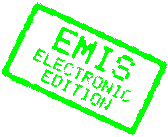The Electronic Library of Mathematics
Mathematical Monographs

For fastest access: Choose your nearest mirror site!

# CURVAS CRITICAS Y SALTOS EN OPTIMIZACION NO LINEAL

by

W. Gomez , J. Guddat, H.Th. Jongen, J.-J. Rückmann

and

C. Solano

Electronic monograph © 2000 J. Guddat and H.Th. JongenThis textbook deals with (one-parametric) nonlinear optimization problems in finite-dimensional Euclidean spaces where the feasible sets under consideration are defined by finitely many (in)equality constraints.

Problems of this type have a wide range of applications.

The main features included in the book are:

• critical point theory (Morse theory) paying attention to topological aspects from both the local and global point of view,
• a generic classification of the local and global structure of the set of generalized critical points of one-parametric nonlinear optimization problems (singularity theory),
• path-following methods with jumps and
• applications of the latter methods, e.g. to embedding algorithms for the solution of nonlinear optimization problems.

The book is divided into eight chapters. The three introductory chapters cover the following concepts:

• local and global minimizer,
• stationary point, Karush-Kuhn-Tucker point,
• (nondegenerate) critical point,
• optimality conditions of first and second order,
• several constraint qualifications which will play a crucial role in topological investigations and
• appropriate differentiable coordinate transformations for local descriptions by normal forms.

Chapter 4 deals with critical point theory for both the unconstrained and the constrained case. Here, the local behaviour of the objective function is studied via normal forms (Morse-Lemma). Moreover, global topological properties of the optimization problem under consideration and, in particular, the existence of critical points with certain characteristic indices are discussed (Morse Relations). These local and global results provide a good insight in topological nonlinear phenomena which can be useful for deriving numerical strategies for solution methods in (global) nonlinear optimization.

Systems of linear inequalities are considered in Chapter 5. Chapters 6, 7 and 8 are concerned with one-parametric nonlinear optimization problems: the describing vector function depends on an additional real parameter. For generic vector functions it turns out that the set of generalized critical points consists of the union of one-dimensional manifolds and can be divided into five disjoint characteristic subsets (five different types of generalized critical points). Chapter 6 presents for each of these five types a local topological and algebraic description.

These descriptions of appearing singularities are basically used for the characterization of pathfollowing methods with jumps. They are established in Chapter 7 under the appropriate assumption that the solution set to be followed (the set of generalized critical points, the set of stationary points or the set of local minimizers) is locally the union of finitely many one-dimensional manifolds. The main idea of path-following methods with jumps is to find solutions for finitely many increasing parameter values from a given parameter interval. If the current curve to be followed has a turning point on this interval, then the method switches locally in a neighbourhood of this turning point to another subset (curve) of the solution set by using a descent algorithm. This latter procedure is called a jump. The existence and characterization of these jumps is discussed for each of the five types of generalized critical points.

Path-following methods have a wide range of applications; they are used for the solution of e. g. nonlinear optimization problems (embedding methods), global optimization problems or problems which depend naturally on a parameter.

In the final Chapter 8 several applications of path-following methods are studied. In particular, exact penalty function methods as well as Lagrange multiplier methods are formulated in the context of one-parametric optimization and it is shown that the concept of path-following with jumps allows a successful application of these classical methods to a wider class of nonlinear optimization problems.

This book serves as a useful reference for both graduate students and researchers in mathematics, economy, operations research and engineering, as well as for optimization practitioners in applied mathematics and engineering who wish to gain a deeper understanding behind the theory of nonlinear optimization.

Although the book is self-contained, the reader is expected to have a basic knowledge in elementary calculus, linear algebra and topology.

Keywords: local and global minimizer, stationary point, Karush-Kuhn-Tucker point, nondegenerate critical point, optimality conditions of first and second order, constraint qualifications, Morse Lemma, linear inequalities, one-parametric nonlinear optimization problems, generic singularities, pathfollowing methods with jumps, embedding methods, penalty function methods, Lagrange mulitplier methods

Classification (MSC2000): Primary: 90C30 Secondary: 90C31, 90C25, 90C26, 90C29

Download the whole book as one file:
[PostScript] (2,765,529 bytes)
[PDF] (2,366,898 bytes)

This book is published exclusively by ELibM
© 2000 ELibM for this page. For the content of the book, the copright remains with the authors.